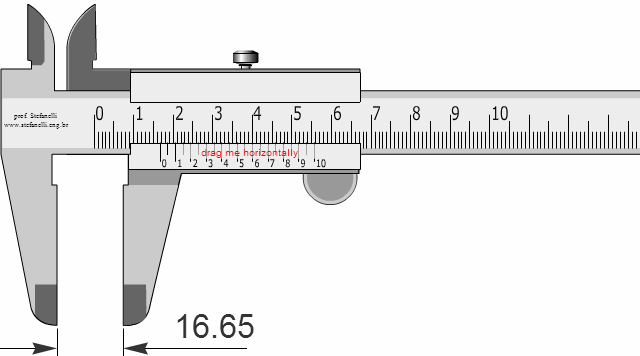# Physics

### Vernier Calliper

Vernier Calliper is a simple device to measure very small lengths easily. The movable scale, also known as Vernier scale, is divided into 10 units. If it slides along the main scale, marked in mm, the gap between two the 0s between the two scales.
If the instrument is accurate, the two zeros must coincide.So,
10 Vernier units = 9 mm
1 Vernier unit = 9/10 mm
1 Vernier unit = 0.9 mm
The gap between 1 unit of the main scale and Vernier unit = 1-0.9 = 0.1 mm
With that, this particular calliper lets us measure lengths which are as small as 0.1 mm.
Therefore, the Vernier scale lets us measure us small lengths. The following animation describes the working of the device.

Let's look at a few of the readings:

E.g.1

Suppose the three different lengths of the red string, read by the main scale, is 4 mm, correct to 1 mm. So its real length is greater than 4 mm and less than 5 mm. The correct value can be calculated as follows:

If 7 mm mark on the main scale coincide with 3th tick of Vernier scale
The value on the Vernier scale = 3x0.9 = 2.7 mm
Therefore, the gap between the two zeros of the scales = 7- 2.7 = 4.3
Length of the red wire = 4.3 mm

E.g.2

If 10 mm mark on the main scale coincide with 6th tick of Vernier scale
The value on the Vernier scale = 6x0.9 = 5.4 mm
Therefore, the gap between the two zeros of the scales = 10-5.4 = 4.6
Length of the red wire = 4.3 mm

E.g.3

If 12 mm mark on the main scale coincide with 8th tick of Vernier scale
The value on the Vernier scale = 8x0.9 = 7.2 mm
Therefore, the gap between the two zeros of the scales = 12-7.2 = 4.8
Length of the red wire = 4.8 mm

So, a certain pattern emerges between the real value and the Vernier reading that coincides with a tick of the main scale.

Vernier ValueReal length
34.3
64.6
84.8
.
.
.
.
.
.
n4+0.1n

If the main scale reading is m and Vernier reading is n, then the real value = m + 0.1n

You can see that we can measure up to 0.1mm, thanks to the above Vernier Scale. Some Vernier Scales are, sometimes, divided into 20 or 30. The more divisions it has, the more accurate it becomes.

E.g.1

If 20 Vernier divisions coincide with 19 mm
20 Vernier units = 19 mm
1 Vernier unit = 19/20 mm
1 Vernier unit = 0.95 mm
The gap between 1 unit of the main scale and Vernier unit = 1-0.95 = 0.05 mm
With that, this particular calliper lets us measure lengths which are as small as 0.05 mm.

If the main scale reading is m and Vernier reading is n, then the real value = m + 0.05n

E.g.2

If 20 Vernier divisions coincide with 29 mm
30 Vernier units = 29 mm
1 Vernier unit = 29/30 mm
1 Vernier unit = 0.97 mm
The gap between 1 unit of the main scale and Vernier unit = 1-0.97 = 0.03 mm
With that, this particular calliper lets us measure lengths which are as small as 0.03 mm.

If the main scale reading is m and Vernier reading is n, then the real value = m + 0.03nThe best book for both teachers and students to learn physics - exactly like in the good old days:concepts are clearly explained in detail;no meaningless cartoons to devour space;the author rendered a great service in his unique approach for generations of students, with this being the fourth edition.

### GCSE Physics - Flash Cards

Amazon Best SellerPhysics flash cards have become an Amazon Best Seller; they are intuitive and summarizes the topic contents really well in beautiful layouts. They are vital for your forthcoming exams.

### A Level Physics - Flash CardsIt's not easy to find flash cards for A Level physics. These cards fill the void with lots of cards, covering the major topics that you need to know. The layout is beautiful and inspiring.